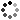# Consumer Math | Simple Interest | FULL Math Version Worksheets |

On Sale
\$5.00
\$5.00
ʕ•́ᴥ•̀ʔっ Greetings!

This download is great for use in solving for balance using the simple interest formula. The questions are scattered throughout the worksheets.

This FULL, No Prep version includes:

✅Worksheets

✅Keys

Questions include:

If you invest (percent) at an interest rate of (rate), how much money will you have after (time)?
You take out a loan for (percent) at an interest rate of (rate) for (time). What is the total amount you will have at the end of (time)?
What will the final balance be for (percent) invested at (rate) for (time)?
If you put (percent) in a savings account that pays (rate) for (time), what is the amount of money you will have at the end of (time)?
If you borrow (percent) at (rate) for (time), how much will you pay back at the end of the term?
You put (percent) into an investment at (rate) for (time). What will the balance be at the end of (time)?

You will get a ZIP (4MB) file
No products found